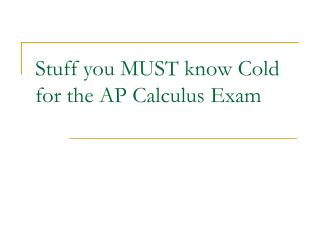# Stuff you MUST know Cold for the AP Calculus Exam - PowerPoint PPT PresentationDownload PresentationStuff you MUST know Cold for the AP Calculus Exam

Stuff you MUST know Cold for the AP Calculus ExamDownload Presentation## Stuff you MUST know Cold for the AP Calculus Exam

- - - - - - - - - - - - - - - - - - - - - - - - - - - E N D - - - - - - - - - - - - - - - - - - - - - - - - - - -
##### Presentation Transcript

1. Stuff you MUST know Cold for the AP Calculus Exam

2. Curve sketching and analysis y = f(x) must be continuous at each: • critical point: = 0 or undefined. • local minimum: goes (–,0,+) or (–,und,+) or > 0 at stationary pt • local maximum: goes (+,0,–) or (+,und,–) or < 0 at stationary pt • point of inflection: concavity changes goes from (+,0,–), (–,0,+), (+,und,–), or (–,und,+)

3. Basic Derivatives

4. Basic Integrals Plus a CONSTANT

5. Some more handy integrals

6. More Derivatives Recall “change of base”

7. Differentiation Rules Chain Rule Product Rule Quotient Rule

8. The Fundamental Theorem of Calculus Other part of the FTC

9. Intermediate Value Theorem • If the function f(x) is continuous on [a, b], and y is a number between f(a) and f(b), then there exists at least one number x = c in the open interval (a, b) such that f(c) = y. Mean Value Theorem . . • If the function f(x) is continuous on [a, b], AND the first derivative exists on the interval (a, b), then there is at least one number x = c in (a, b) such that

10. Mean Value Theorem & Rolle’s Theorem If the function f(x) is continuous on [a, b], AND the first derivative exists on the interval (a, b), then there is at least one number x = c in (a, b) such that If the function f(x) is continuous on [a, b], AND the first derivative exists on the interval (a, b), AND f(a) = f(b), then there is at least one number x = c in (a, b) such that f '(c) = 0.

11. Extreme Value Theorem If the function f(x) is continuous on [a, b], then f has both an absolute maximum and an absolute minimum on [a,b] The absolute extremes occur either at the critical points or at the endpoints.

12. Approximation Methods for Integration Riemann Sum LRAM when ck is a LEFT endpoint RRAM when ck is a RIGHT endpoint MRAM when ck is a MIDPOINT Trapezoidal Rule

13. Theorem of the Mean Valuei.e. AVERAGE VALUE • If the function f(x) is continuous on [a, b] and the first derivative exists on the interval (a, b), then there exists a number x = c on (a, b) such that • This value f(c) is the “average value” of the function on the interval [a, b].

14. Solids of Revolution and friends • Arc Length • Disk Method • WasherMethod • General volume equation (not rotated)

15. Distance, Velocity, and Acceleration velocity = (position) average velocity = (velocity) acceleration = speed = velocity vector = displacement =

16. Values of Trigonometric Functions for Common Angles π/3 = 60° π/6 = 30° θ sin θ cos θ tan θ 0° 0 1 0 sine ,30° cosine ,45° 1 ,60° ,90° 1 0 ∞ π,180° 0 –1 0

17. Trig Identities Double Argument Pythagorean sine cosine

18. Slope – Parametric & Polar Parametric equation • Given a x(t) and a y(t) the slope is Polar • Slope of r(θ) at a given θ is What is y equal to in terms of r and θ ? x?

19. Polar Curve For a polar curve r(θ), the AREA inside a “leaf” is (Because instead of infinitesimally small rectangles, use triangles) where θ1 and θ2 are the “first” two times that r = 0. We know arc length l = rθ and

20. l’Hôpital’s Rule If then

21. Other Indeterminate forms: Write as a ratio Use Logs

22. Integration by Parts We know the product rule Antiderivative product rule (Use u = LIPET) e.g. L I P E T Logarithm Inverse Polynomial Exponential Trig Let u = ln x dv = dx du = dx v = x

23. Maclaurin SeriesA Taylor Series about x = 0 is called Maclaurin. Taylor Series If the function f is “smooth” at x = a, then it can be approximated by the nth degree polynomial